Get instant live expert help with Excel or Google Sheets“My Excelchat expert helped me in less than 20 minutes, saving me what would have been 5 hours of work!”

#### Post your problem and you’ll get expert help in seconds.

Your message must be at least 40 characters
Our professional experts are available now. Your privacy is guaranteed.

# Working with a Calculated Field in an Excel Pivot Table

In Excel, Pivot table Calculated Fields can be added as new fields in a Pivot table. These contain values based on calculations performed on data from Pivot table field(s). Calculated Fields do not contain any data themselves, but these fields derive data based on formula calculations on Pivot table field(s).

## Calculated fields in Excel Pivot Tables

Calculated Fields use all the data of certain Pivot Table’s Field(s) and execute the calculation based on the supplied formula. Calculated Fields can add/ subtract/multiply/divide the values of already present data fields. In this article, you will learn how to create, modify and delete a Calculated Field in a Pivot table.

Let’s assume you are working in a company who sells different brands of cigarettes in different regions. You have a dataset of Sales that contains data fields of Region, Brand, Quantity Sold, Unit Price and Sales Amount.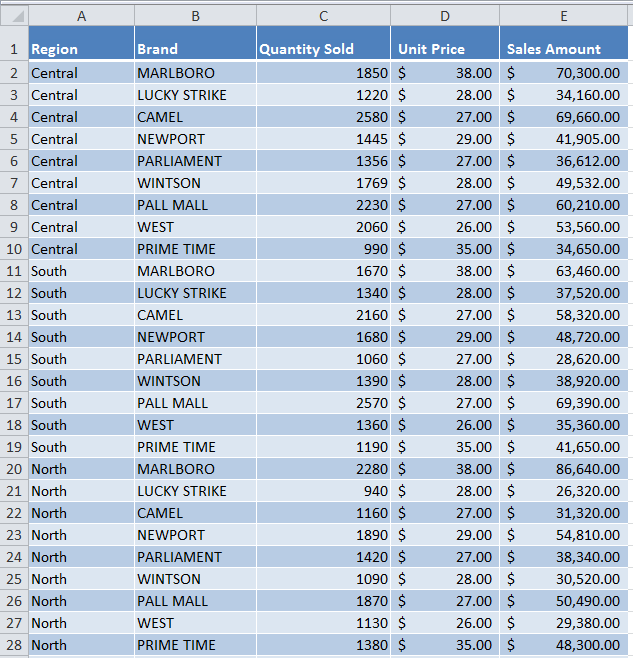Using a Pivot table, you can easily summarize sales data of region and brand fields by quantity sold and sales amount by placing Region and Brand fields in Row area, and Quantity Sold and Sales Amount fields in Values area as shown below.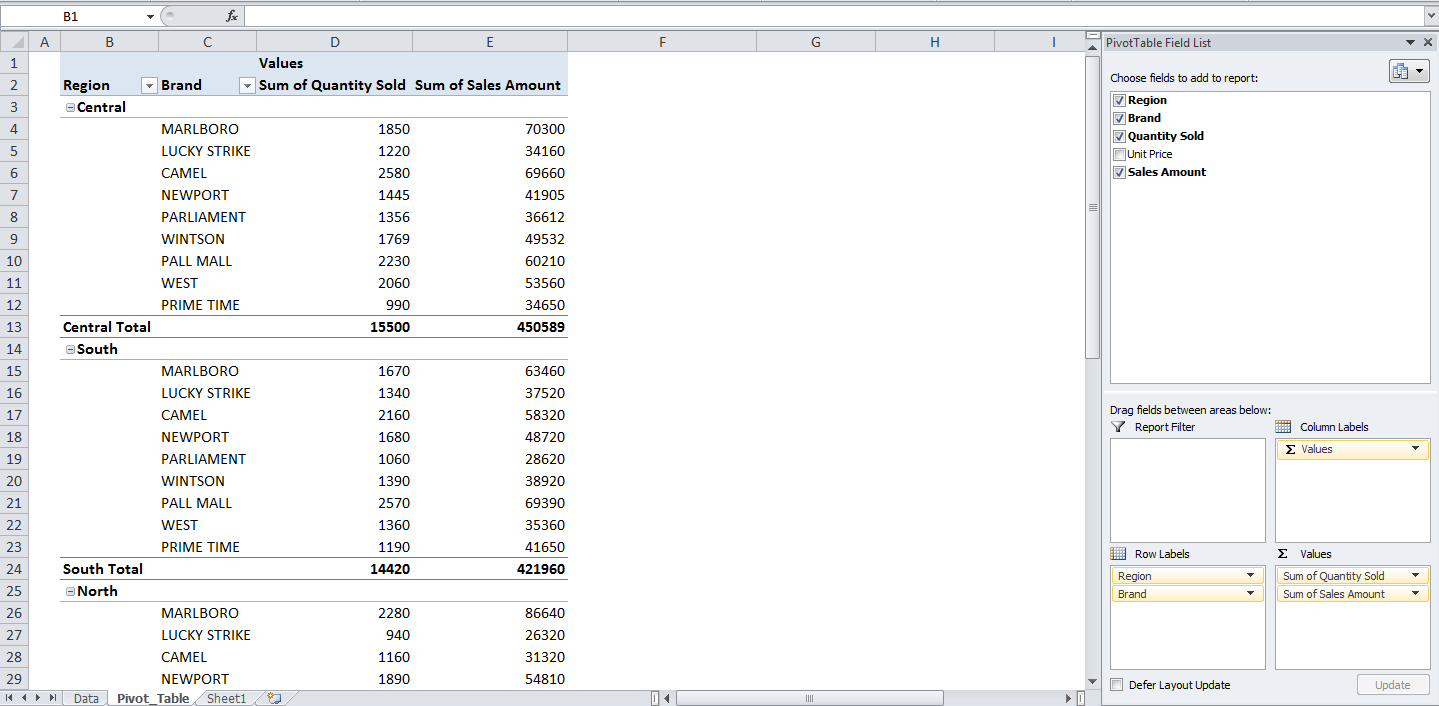Now you want to calculate and summarize Cost of Goods Sold and Gross Profit in a Pivot table. In this example, you will learn how to create/add these new Calculated Fields by using the data of other fields in a Pivot table based on a formula.

You can calculate Cost of Goods Sold and Gross Profit by applying the following formulas;

`Cost of Goods Sold= Sales Amount * 60%`

You can calculate values of Cost of Goods Sold by multiplying values of Sales Amount field by a constant of 60%.

`Gross Profit= Sales Amount – Cost of Goods Sold`

You can calculate the values of Gross Profit field by subtracting the values of Cost of Goods Sold field from values of Sales Amount field.

### How to create a Calculated Field in a Pivot Table

Now you will learn how to create these Calculated Fields one by one by following these steps. To insert a Calculated Field, execute the following steps.

• Click any cell inside the pivot table.
• On Options or Analyze tab, in the Calculations group, click Fields, Items & Sets and click Calculated Field.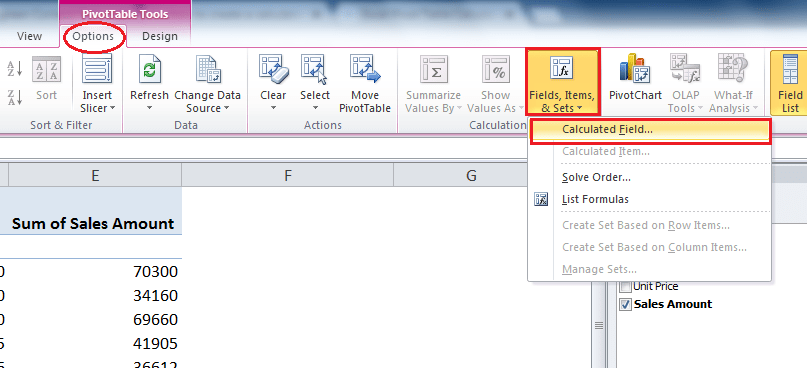• The Insert Calculated Field dialog box appears.
1. Enter Name of Calculated Field
2. Type the formula
4. Click OK button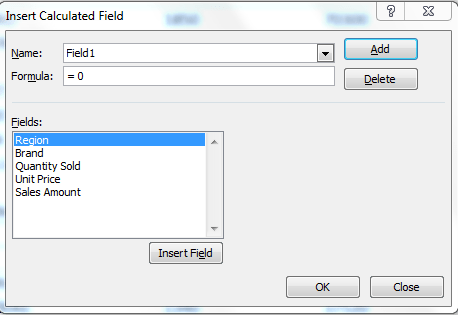Now, by following the above steps, you will learn to create your desired two Calculated Fields as discussed above.

### Cost of Goods Sold Calculated Field

This calculated field uses the following Pivot table field in the below formula;

`Formula = ‘Sales Amount’ * 60%`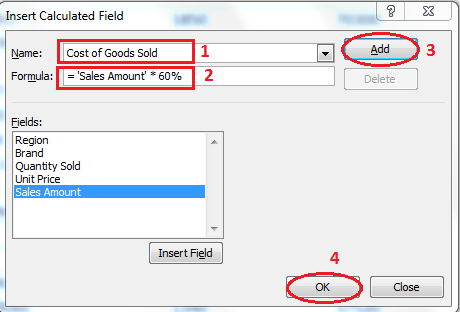Excel automatically creates this Calculated Field and adds in Values area of Pivot Table Fields List panel. As this field contains numbers, so Pivot table by default SUM the values, as shown below;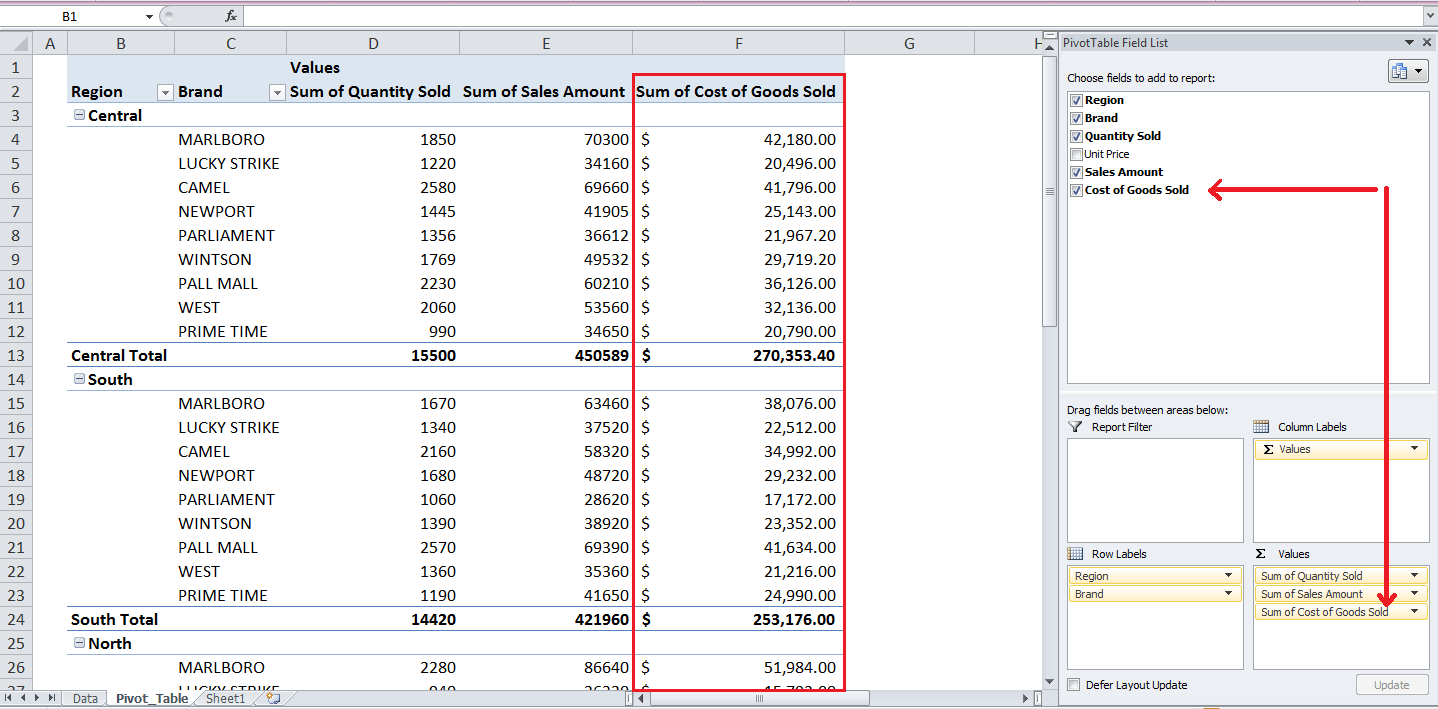### Gross Profit Calculated Field

This calculated field uses the following fields in the below formula;

`Formula = ‘Sales Amount’ – ‘Cost of Goods Sold’`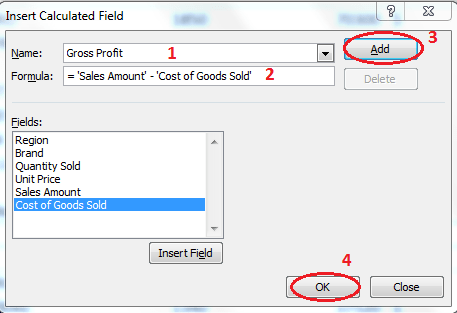Calculated Field is created automatically and added to Pivot table Fields list’s Values area, and resulting values are summarized by SUM.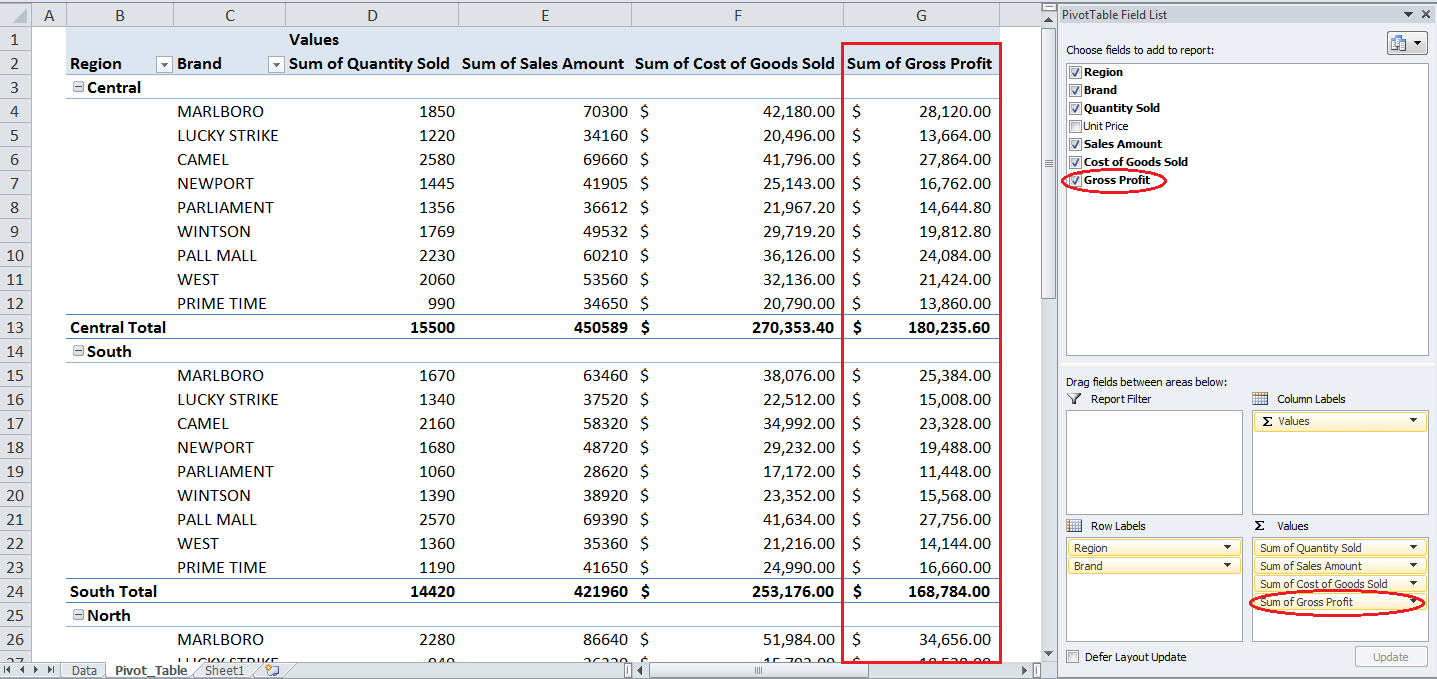### How to modify Calculated Fields in a Pivot Table

You can modify an existing Calculated Field by editing its formula in Insert Calculated Field dialog box by following these steps;

1. Click drop down the list of Name, select Calculated Field name you want to modify
2. Edit the formula
3. Click on Modify button
4. Click OK button

Now suppose you want to modify the Cost of Goods Sold calculated field by editing the percentage in formula from 60% to 55%. By following the above steps, you can modify this existing Calculated Field, and its values will be updated automatically. This change will show the impact on calculations of other Calculated Fields, where this Calculated Field is used, such as in Gross Profit.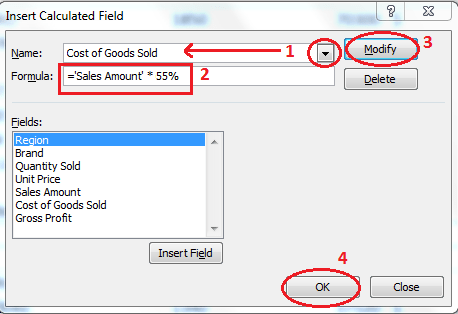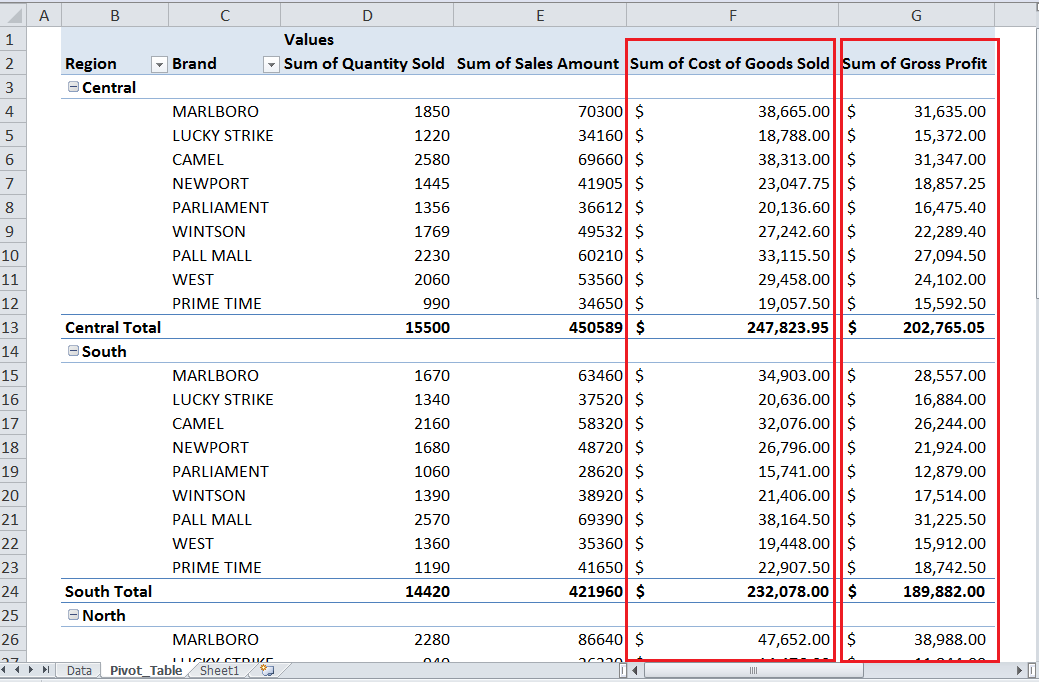### How to delete a Calculated Fields in Pivot Table

You can delete a Calculated Field from Pivot table by performing the following steps on Insert Calculated Field dialog box;

1. Click drop down the list of Name, select Calculated Field name you want to delete
2. Click on Delete button
3. Click OK button

Suppose you want to delete Gross Profit Calculated Field from Pivot table, so you can do it by following the above steps, as shown below.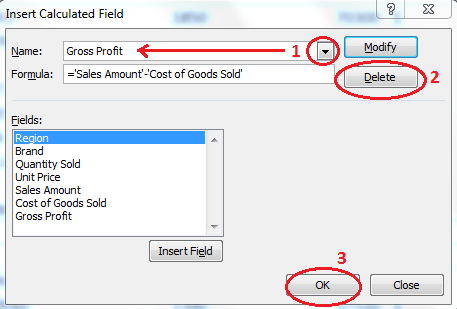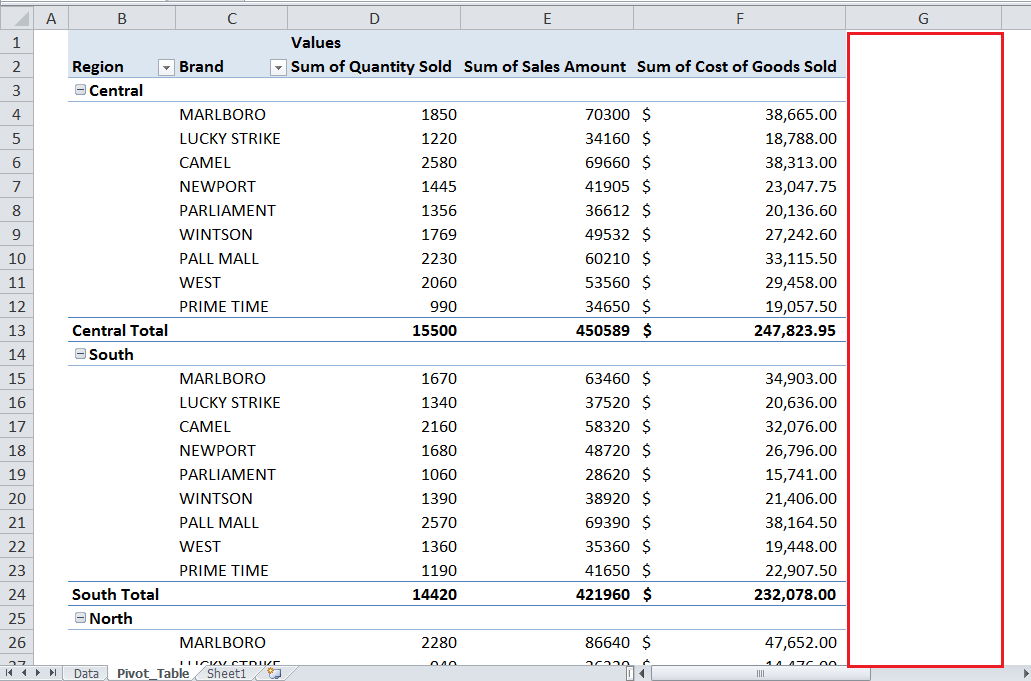Still need some help with Excel formatting or have other questions about Excel? Connect with a live Excel expert here for some 1 on 1 help. Your first session is always free.

### Did this post not answer your question? Get a solution from connecting with the expert.Another blog reader asked this question today on Excelchat:
Related blogs
Solution examplesI need a formula to pop at the upper left corner of a spreadsheet. If I enter the month "January," I want the column number sum of January =SUM(AB11:AB75) from another section on the same excel page to pop right below the "January" cell, and not display the formula expression, but see the \$100.
Solved by T. Q. in 40 minsCan't add (SUM) in imported numbers from bank account
Solved by F. C. in 40 minsI need a formula to combine D2 to D100 to add together a column of numbers, then take away the same amount on the same row when column E is filled. i.e. column D is a price of an item, so the formula must calculate the total, then when the item is sold an 'a' is marked next to the item in column E, the formula then must deduct this amount from the total
Solved by X. W. in 20 minsI would like to have a diagram in a new sheet, where the horizontal axis is the days, as they are in column DX. Each day shall show the sum of all unique leads of that day, and I would like to be able to check via a box of checkboxes, which facilities are shown, the facilities are in column BC.
Solved by I. A. in 45 minsI am working on a cash flow projection. Part of the projection includes sales commissions. Our sales guys earn a monthly draw and then commission on sales after a certain amount. For example they may earn a monthly salary of 12,500 and earn additional commission after their commission equals \$150,000. What I need excel to do is sum a column if the values in the preceeding columns are greater than \$150,000. I've tried using the sumif and the if function in excel and it's not working correctly either way. Any suggestions?
Solved by G. W. in 19 mins## Subscribe to Excelchat.coAnother blog reader asked this question today on Excelchat: